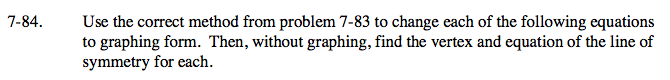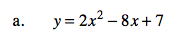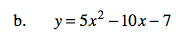### Home > A2C > Chapter 7 > Lesson 7.1.5 > Problem7-84

7-84.Complete the square to find the equation.

y = 2(x2 − 4x + 4) + 7 − 8

Use y = a(xh)2 + k and (h, k) to find the vertex and axis of symmetry.

y = 2(x − 2)2 − 1

vertex: (2, −1)
axis of symmetry: x = 2See part (a).

y = 5(x − 1)2 − 12
vertex: (1, −12)
axis of symmetry: x = 1